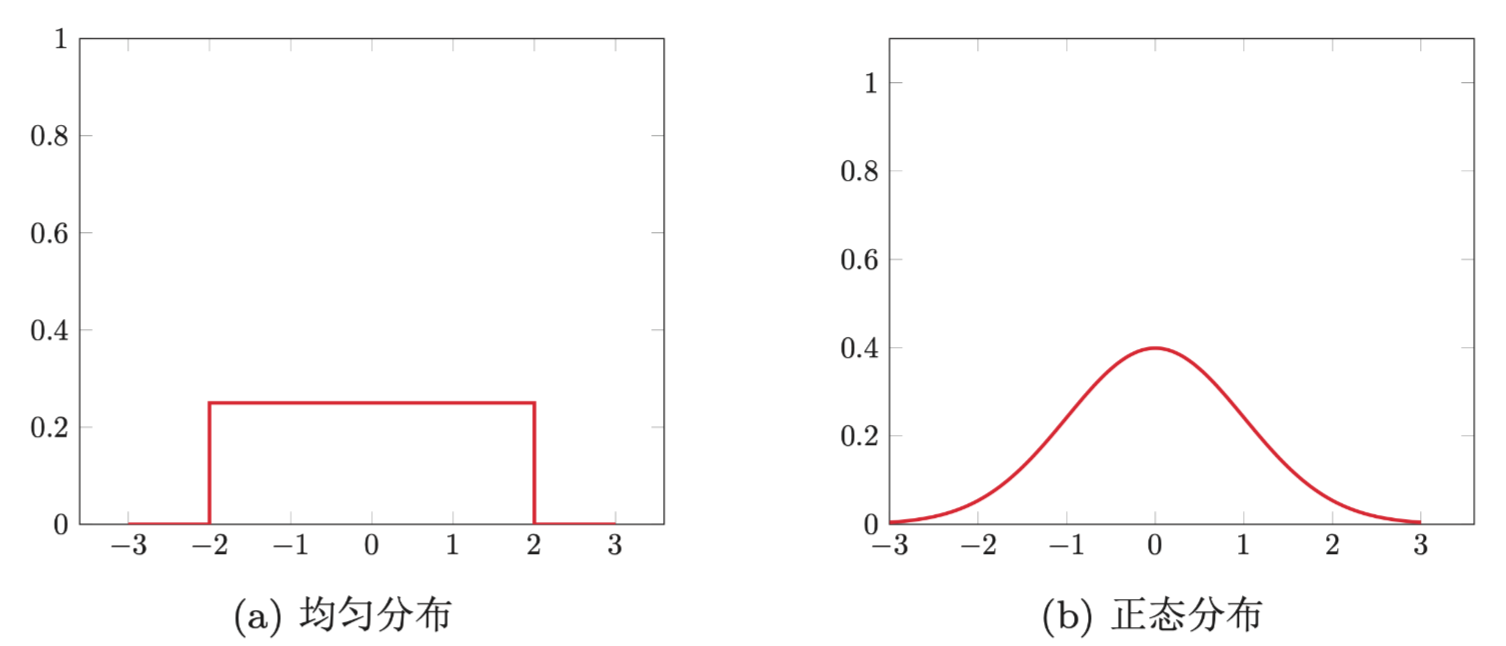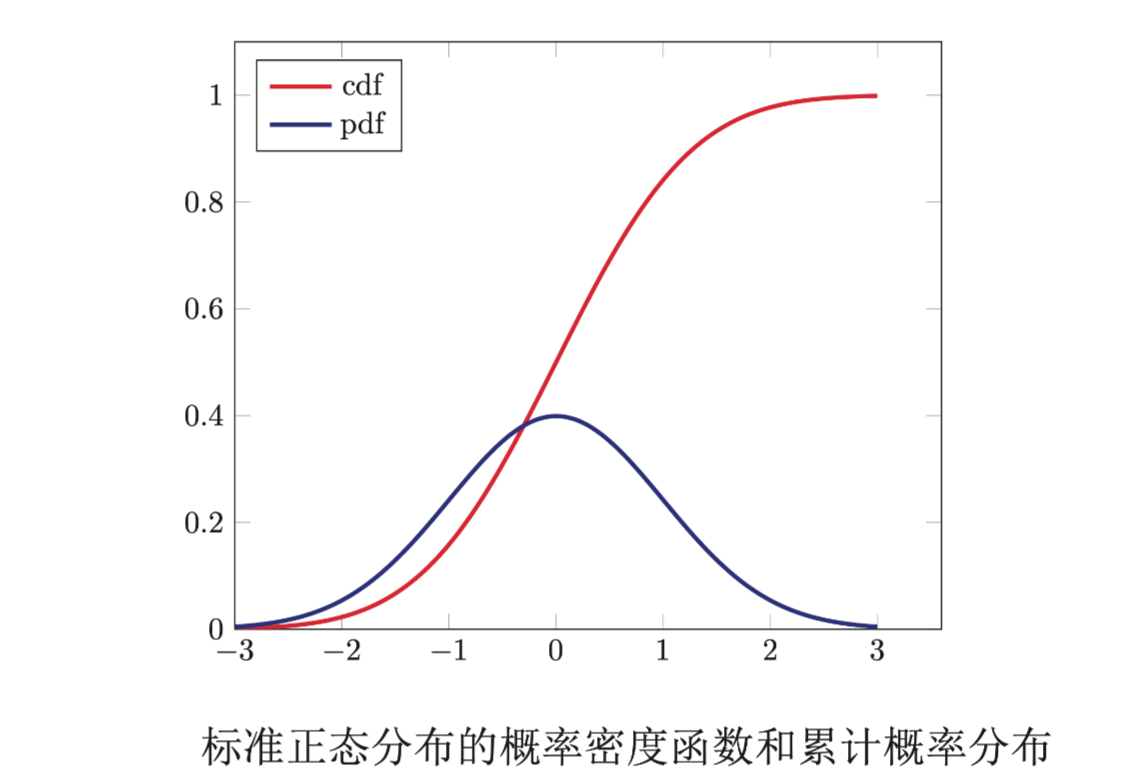【算法工程师的数学基础】系列将会从线性代数、微积分、数值优化、概率论、信息论五个方面进行介绍，感兴趣的欢迎关注【搜索与推荐Wiki】公众号，获得最新文章。

《算法工程师的数学基础》已更新：

## 样本空间

• 总体方差（variance）：总体中变量离其平均值距离的平均。比如一组数据$x_1, x_2, …, x_n$，则其方差表达式为：

• 样本方差（variance）：样本中变量离其平均值距离的平均。比如一组样本数据为$x_1, x_2, …, x_n$，则其方差表达式为：

## 事件和概率

• {正面}：概率为0.5
• {负面}：概率为0.5
• 空集：即不是正面，也不是负面，概率为0
• {正面 | 负面}：正面或者负面，概率为1

### 随机变量

#### 离散型随机变量

$p(x_1),…,p(x_n)$称为离散型随机变量$X$的概率分布（Probability Distributuin）或分布，并且满足：

• 伯努利分布
• 二项分布

#### 连续型随机变量

• 均匀分布
• 正态分布#### 累积分布函数Dirichlet分布

### 边际分布

（1）对于固定的$x$：$\sum_{y \in \Omega_y} p(x,y)=P(X=x)=p(x)$

（2）对于固定的$y$：$\sum_{x \in \Omega_x} p(x,y)=P(Y=y)=p(y)$

## 随机过程

### 高斯过程

【技术服务】，详情点击查看： https://mp.weixin.qq.com/s/PtX9ukKRBmazAWARprGIAg